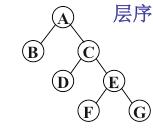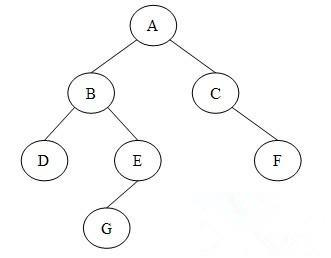• 二叉树层序遍历C语言
2022-03-18 22:44:11

# 二叉树层序遍历C语言版

leetcode 102

/**
* Definition for a binary tree node.
* struct TreeNode {
*     int val;
*     struct TreeNode *left;
*     struct TreeNode *right;
* };
*/

/**
* Return an array of arrays of size *returnSize.
* The sizes of the arrays are returned as *returnColumnSizes array.
* Note: Both returned array and *columnSizes array must be malloced, assume caller calls free().
*/

int** levelOrder(struct TreeNode* root, int* returnSize, int** returnColumnSizes){
* returnSize = 0;
* returnColumnSizes = malloc(sizeof(int) * 2010);
if(root == NULL){
return 0;
}
int i = 0;
struct TreeNode* node;
int front=0, top = 0;
struct TreeNode** q = malloc(sizeof(struct TreeNode*) * 2010);
int** ret = malloc(sizeof(int*) * 2010);
q[top++] = root; //q = root
// 队列为空 top==front
while(top - front > 0){

int q_size = top - front;
(* returnColumnSizes)[i] = q_size;
ret[i] = malloc(sizeof(int) * q_size);
for(int j =0; j < q_size;j++){
node = q[front++]; //出队
ret[i][j] = node->val;
if(node->left != NULL)  q[top++]= node->left;     // push
if(node->right != NULL)  q[top++]= node->right;
}
i++;
}
* returnSize = i;
return ret;

}


挺有意思的

更多相关内容
• 贴代码： #include<...//定义二叉树 typedef struct BiNode { char data; struct BiNode* lchild, * rchild; }BiNode,*BiTree; //定义循环队列 typedef struct { BiTree data[MAXSIZE]; int fr

贴代码：

#include<stdio.h>
#include<stdlib.h>

#define OK 1
#define ERROR 0
#define MAXSIZE 10

//定义二叉树
typedef struct BiNode {
char data;
struct BiNode* lchild, * rchild;
}BiNode,*BiTree;

//定义循环队列
typedef struct {
BiTree data[MAXSIZE];
int front;
int rear;
}Queue;

//初始化队列
int InitQueue(Queue* Q) {
Q->front = 0;
Q->rear = 0;
return OK;
}
//插入队列
int EnQueue(Queue* Q, BiTree bt) {
if ( (Q->rear+1)%MAXSIZE==Q->front )
{
return ERROR;
}
Q->data[Q->rear] = bt;
Q->rear = (Q->rear + 1)%MAXSIZE;
return OK;
}

//删除队列  注意这里的第二个形参实际上用了二级指针
int DeQueue(Queue* Q, BiTree* bt) {
if (Q->front==Q->rear)
{
return ERROR;
}
(*bt) = Q->data[Q->front];
Q->front = (Q->front + 1) % MAXSIZE;
return OK;
}

//二叉树的创建
void CreateBiTree(BiTree* T) {
char c;
scanf_s("%c", &c);
if (c==' ')
{
(*T) = NULL;
}
else
{
(*T) = (BiTree)malloc(sizeof(BiNode));
(*T)->data = c;
CreateBiTree(&(*T)->lchild);
CreateBiTree(&(*T)->rchild);
}
}

//层序遍历二叉树实现
void LevelOrder(BiTree T) {
if (T)
{
Queue Q;
InitQueue(&Q);
EnQueue(&Q, T);
BiTree bt = NULL;
while (Q.front!=Q.rear)
{
DeQueue(&Q, &bt);
printf("%c ", bt->data);
if (bt->lchild)
{
EnQueue(&Q, bt->lchild);
}
if (bt->rchild)
{
EnQueue(&Q, bt->rchild);
}
}
}
}

//主函数测试
int main() {
BiTree T = NULL;
CreateBiTree(&T);
LevelOrder(T);
}

测试结果：
输入： A_ _BD_ _E_ _CF_ _G_ _  ，这里下划线代表空格（测试二叉树在下面）
输出结果： A B C D E F G展开全文• ## c语言实现二叉树层序遍历

万次阅读 多人点赞 2018-08-11 21:19:11
层序遍历原则，应打印ABCDEFG，如何实现？ 1.使用队列，队列是先进先出，首先把A放进去，然后如果队列有元素，就出队A，然后把出队元素A的左右BC节点入队，然后B出队，把B的左右节点放进去（没有就继续出队C），C...按层序遍历原则，应打印ABCDEFG，如何实现？

1.使用队列，队列是先进先出，首先把A放进去，然后如果队列有元素，就出队A，然后把出队元素A的左右BC节点入队，然后B出队，把B的左右节点放进去（没有就继续出队C），C出队，把DE放进去，D出队，E出队，把FG放进去,然后出FG（因为FG左右节点没有数据,不用入队）,循环条件是队列不能为空（才能实现出队操作）

核心源码:

void LevelOrderBinaryTree(pTreeNode t){
pQueue pq=(pQueue)malloc(sizeof(Queue));
pq=init(pq);
enqueue(pq,t);
while(pq->rear!=pq->front){
pTreeNode x=dequeue(pq);
printf("%d ",x->data);
if(x->left){
enqueue(pq,x->left);
}
if(x->right){
enqueue(pq,x->right);
}
}
}

注意：(1).队列不为空即front不等于rear

(2).逻辑要缜密，要出队，实现队列不能为空是把，要入队，首先入队元素不能为空是把

(3).入队后出队，出队要把元素输出（data），然后要把该元素的left，right节点入队，所以要把pTreeNode节点存进去，出队返回该树节点，然后输出该节点的数据，最后把他的左右节点入队

(4).声明结构体，最好多加个结构体指针，在函数传入，只需4个字节，提高效率，不用把整个结构体传进去

完整代码如下：

#include<stdio.h>
#include<stdlib.h>
#include<malloc.h>
typedef struct TreeNode{
TreeNode *left;
TreeNode *right;
int data;
}TreeNode,*pTreeNode;

typedef struct QueueNode{
pTreeNode data;
QueueNode *next;
}QueueNode,*pQueueNode;

typedef struct Queue{
pQueueNode front;
pQueueNode rear;
}Queue,*pQueue;

void create(pTreeNode *t){
int ch;
scanf_s("%d",&ch);
if(ch==-1){
(*t)=NULL;
return;
}else{
(*t)=(pTreeNode)malloc(sizeof(TreeNode));
(*t)->data=ch;
printf("请输入%d的左节点数据:",ch);
create(&((*t)->left));
printf("请输入%d的右节点数据:",ch);
create(&((*t)->right));
}
}

pQueue init(pQueue pq){
pq->front=(pQueueNode)malloc(sizeof(QueueNode));
pq->front->next=NULL;
pq->rear=pq->front;
return pq;
}

void enqueue(pQueue pq,pTreeNode t){
pQueueNode pNew=(pQueueNode)malloc(sizeof(QueueNode));
pNew->data=t;
pNew->next=NULL;
pq->rear->next=pNew;
pq->rear=pNew;
}

pTreeNode dequeue(pQueue pq){
pQueueNode pTemp=(pQueueNode)malloc(sizeof(QueueNode));
pTemp=pq->front->next;
if(pTemp->next==NULL){
pq->rear=pq->front;
}else{
pq->front->next=pTemp->next;
}
pTreeNode x=pTemp->data;
free(pTemp);
return x;
}

void LevelOrderBinaryTree(pTreeNode t){
pQueue pq=(pQueue)malloc(sizeof(Queue));
pq=init(pq);
enqueue(pq,t);
while(pq->rear!=pq->front){
pTreeNode x=dequeue(pq);
printf("%d ",x->data);
if(x->left){
enqueue(pq,x->left);
}
if(x->right){
enqueue(pq,x->right);
}
}
}
void main(){
pTreeNode t;
printf("请输入第一个节点数据,-1代表没数据:");
create(&t);
system("pause");
printf("层序遍历如下:");
LevelOrderBinaryTree(t);
system("pause");
}

展开全文数据结构
• ## 二叉树层序遍历（C语言）

千次阅读 多人点赞 2021-04-25 22:14:28
二叉树层序遍历即从上到下，在每一层从左到右依次打印数据。 如下： 层序遍历结果： ABCDEFG 基本思路即将根节点入队后，之后每次都将队首元素出队，打印队首元素数据，并将队首元素左右子树入队，一直重复上述...

二叉树的层序遍历即从上到下，在每一层从左到右依次打印数据。

如下：层序遍历结果：
ABCDEFG

基本思路即将根节点入队后，之后每次都将队首元素出队，打印队首元素数据，并将队首元素左右子树入队，一直重复上述过程。

自然，本题还可以用数组来实现。

代码：

#include <stdio.h>
#include <stdlib.h>
#define QueueMax 100

typedef struct Node
{
char data;
struct Node *LChild, *RChild;
}BiNode, *BiTree;

typedef struct
{
BiTree data[QueueMax];
int rear;
int len;
}Queue;

BiTree CreateTree();  //建立二叉树
Queue InitQueue();  //初始化队列
int IsEmptyQueue(Queue seq);  //队列判空
int IsFullQueue(Queue seq);   //队列判满
void PushQueue(Queue *seq, BiTree T);  //入队
void PopQueue(Queue *seq, BiTree *T);  //出队
void LayerOrder(BiTree T);  //层序遍历

int main()
{
BiTree T;
T = CreateTree();
LayerOrder(T);
return 0;
}

BiTree CreateTree()
{  //建立二叉树
char c;
c = getchar();
BiTree T;
if (c == '#') {
return NULL;
}
T = (BiTree) malloc (sizeof(BiNode));
T->data = c;
T->LChild = CreateTree();
T->RChild = CreateTree();
return T;
}

Queue InitQueue()
{  //初始化队列
Queue seq;
for(int i = 0; i < QueueMax; i++) {
seq.data[i] = NULL;
}
seq.rear = -1;
seq.len = 0;
return seq;
}

int IsEmptyQueue(Queue seq)
{  //队列判空
if (seq.len == 0) {
return 1;
}
return 0;
}

int IsFullQueue(Queue seq)
{  //队列判满
if (seq.len == QueueMax) {
return 1;
}
return 0;
}

void PushQueue(Queue *seq, BiTree T)
{  //入队
if (IsFullQueue(*seq)) {
printf("ErrorFull");
return;
}
seq->rear = (seq->rear + 1) % QueueMax;
seq->len++;
seq->data[seq->rear] = T;
}

void PopQueue(Queue *seq, BiTree *T)
{  //出队
if (IsEmptyQueue(*seq)) {
printf("ErrorEmpty");
return;
}
seq->len--;
}

void LayerOrder(BiTree T)
{  //层序遍历
Queue seq;
seq = InitQueue();
BiTree tmp;
tmp = T;
PushQueue(&seq, tmp);
while(!IsEmptyQueue(seq)) {
printf("%c", tmp->data);
if (tmp->LChild != NULL) {
PushQueue(&seq, tmp->LChild);
}
if (tmp->RChild != NULL) {
PushQueue(&seq, tmp->RChild);
}
PopQueue(&seq, &tmp);
}
}


展开全文队列 数据结构
• ## C语言层序遍历二叉树（递归）

千次阅读 多人点赞 2021-01-06 18:30:58
层序遍历意思就是按照从上往下，从左往右的顺序遍历二叉树的元素。 实现二叉树层序遍历需要用到二叉树和队列。 总体思路： 判断当前队列是否为空 队列为空：结束。队列非空：取出队列第一个元素 将取出的元素的两...队列 数据结构 算法
• 顾名思义，层序遍历就是把二叉树按层遍历，一层一层从根节点往深度方向遍历，每到新的一层，就把该层的所有节点遍历一遍，再进入下一层。 举个栗子⑧： 我们将下图中的二叉树划分为3层，并给每个节点按层从上到下，...算法
• 二叉树遍历及应用(栈和队列实现二叉树遍历) 案例树 代码 #include <stdio.h> #include <malloc.h> #include <stdlib.h> #define OK 1 #define ERROR 0 typedef int Status; //（1）二叉树...数据结构 链表 算法 树结构
• } } //层序遍历二叉树深度 int LevelDepth(BiTree T) { int h;//暂存当前访问到的层次 if (!T) h = 0;//空树 else { LinkQueue Q; ElemType p; InitQueue(Q);//初始化队列 T->level = 1;//根的层序1 ...数据结构
• 对于二叉树来说，它是一个递归的定义，我们要实现层次遍历必然要满足从上到下、从左到右这个要求，从根结点出发，我们可以将所有意义上的根结点都存储在队列之中，那我们可以使用队列先进先出的特点来实现要求的遍历...算法 数据结构
• 本实验通过实现二叉树的链式存储实现及二叉树的基本操作，掌握递归算法的 设计、递归算法与非递归算法之间的转换和遍历技术，为后续章节学习图的内容奠 定基础。 二、实验内容 (1)、以链表为存储结构创建二叉树； (2...数据结构 链表
• } 利用队列，对二叉树进行层序遍历，代码直接copy可以运行，无bug. 队列使用的链式队列，没有使用数组作为队列。 输出结果为： 1 2 3 4 5 6 7 8 9 10 11 12 上述也实现了前序、中序、后序遍历 函数的申明如下： ...算法 数据结构
• 1.创建二叉树结点和值 class Node: def __init__(self, value): self.value = value self.left = None self.right = None 2.构造二叉树 alist = [1, 2, 3, 4, 5, 6, 7, 8, 9] def creatTree(ali...数组
• 二叉树的遍历分为前序、中序、后序以及层序遍历。 前中后序是按照根结点的访问顺序来确定的，前中后序遍历的核心都是递归。 一、前序遍历 访问顺序：根->左->右 普通二叉树遍历（前序）：ABDCG 扩展...数据结构 算法 c++ 链表
• 二叉树层序遍历的结果是 [ , [9,20], [15,7] ] 代码： public class BinaryTreeLevelOrder { public static class TreeNode { int val = 0; TreeNode left = null; TreeNode right = null; public ...队列 java 算法
• 对于深度为 D 的，有 N 个结点的二叉树，若其结点对应于相同深度完美二叉树层序遍历的前 N 个结点，这样的树就是完全二叉树。 给定一棵完全二叉树的后序遍历，请你给出这棵树的层序遍历结果。 输入格式： 输入在第...算法 数据结构
• 欢迎大家交流指正 #include<stdio.h> #include<stdlib.h> #define MAXSIZE_QUEUE 1000 #define MAXSIZE_STACK 1000 typedef char TElemType;... //非递归后续遍历使用,用于统计次数数据结构
• 采用C语言二叉树的前序、中序、后序、层序（使用队列）遍历方法进行了实现，含一个.c文件和一个.h文件，程序的结构比较清晰，对学习二叉树和队列的相关技术具有一定参考意义（有问题可留言交流）
• 二叉树层序遍历题目思路103.二叉树的锯齿形层序遍历题目199.二叉树的右视图题目：代码 二叉树的层序遍历（bfs） 思想： 二叉树的层序遍历主要是运用bfs的思想，进行一层一层的遍历 BFS: 创建队列Queue,然后从当前一...java bfs
• C语言用递归法将二叉树层序遍历，并求出最大宽度。文件类型是.cpp的，c的编译器都可以编译。
• #include #include/*** 二叉树二叉链表之非递归遍历：层序遍历* 算法思想：借助一个队列；根树进队；队不为空时循环“从队列中出一个树p，访问该树根结点；* 若它有左子树，左子树进队；若它有右子树，右子树进队。...
• ps：2018/7/15自己花了一晚上的时间写的二叉树层序遍历，但是感觉有点小瑕疵，各位大佬帮忙看看#ifndef _Moni_H#define _Moni_Hstruct cenxu;struct queue;typedef struct cenxu * abc;typedef struct queue * adf...c/c++
• 二叉树层序遍历 我们回归正题，什么是二叉树层序遍历，就是将二叉树分层，每一层从左至右输出，如图所示 输出结果为 乍眼一看和广度优先搜索（BFS）没什么区别，但是层序遍历要求输出结果与BFS不同，层序遍历...数据结构 queue java leetcode
• 给定一个二叉树，返回该二叉树层序遍历的结果，（从左到右，一层一层地遍历） 例如： 给定的二叉树是{3,9,20,#,#,15,7}, 如果你不清楚“{1,#,2,3}"的含义的话，请继续阅读 我们用如下方法将二叉树序列化： ...数据结构 java 队列 算法
• 二叉树的定义 ...二叉树有三种遍历方式，分别为先序遍历、中序遍历、后序遍历 先序遍历： （1）先访问根节点。 （2）再访问左子树。 （3）最后访问右子树。 中序遍历： （1）先访问左子树。 .数据结构 开发语言
• C语言实现二叉树遍历极其应用〔摘要〕：《数据结构》是计算机系学生的一门专业技术基础课程，计算机科学各领域及有关的应用软件都要用到各种数据结构。C语言有较丰富的数据类型、运算符以及函数，能直接与...
• 对于深度为 D 的，有 N 个结点的二叉树，若其结点对应于相同深度完美二叉树层序遍历的前 N 个结点，这样的树就是完全二叉树。 给定一棵完全二叉树的后序遍历，请你给出这棵树的层序遍历结果。 输入格式： 输入在第...数据结构
•  Binary Tree Level Order Traversal一、问题描述给定一个二叉树，返回其节点值的层序遍历。（即从左到右，逐级）【举例】给定一个二叉树 [3,9,20,null,null,15,7], 3 / \ 9 20 / \ 15 7返回层序遍历:[ ,...
•数据结构 链表
• 107. 二叉树层序遍历 II1. 题目描述2.代码如下 1. 题目描述 给定一个二叉树，返回其节点值自底向上的层序遍历。 （即按从叶子节点所在层到根节点所在的层，逐层从左向右遍历） 例如： 给定二叉树 [3,9,20,null,...
• 层序遍历就是按二叉树一层一层遍历，我认为已经很直观了其实。写这篇博客就是加强我二叉树与栈和队列的联合应用的能力，毕竟学到道理与敲代码出来差距是很大的。 #include<stdio.h> #include<stdlib.h> ...队列 数据结构 算法...